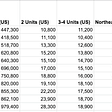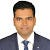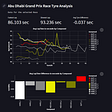# Goal of Analysis

The goal of this project is to use these features in order to make predictions if a patient has cardiovascular disease. Classification models like Logistics Regression will be used in this dataset.

# Datasets

We will be training and testing our datasets and predict the outcomes. Here we get datasets https://www.kaggle.com/sulianova/cardiovascular-disease-dataset

# Data Exploration

Exploration and modeling will be conducted using a Jupyter notebook. We begin by loading the required libraries and importing the data as a DataFrame.

`import numpy as npimport pandas as pdimport matplotlib.pyplot as pltimport seaborn as sns%matplotlib inlineimport warningswarnings.filterwarnings('ignore')df = pd.read_csv("Downloads\cardio_train.csv", sep=";")df.head()`

The data frame has 13 columns and 69301 observations. No missing values detected. 1 feature is float type and rest are int. It means that all the features are numerical.

# Exploratory Data Analysis

`Convert age from days to years and let's see how our data is varyingfrom matplotlib import rcParamsrcParams['figure.figsize'] = 9, 6df['years'] = (df['age'] / 365).round().astype('int')sns.countplot(x='years', hue='cardio', data = df, palette="Set2");`

Let’s look at categorical variables in the dataset and their distribution

`df_categorical = df.loc[:,['cholesterol','gluc', 'smoke', 'alco', 'active']]sns.countplot(x="variable", hue="value",data= pd.melt(df_categorical))`

Bivariate analysis

It may be useful to split categorical variables by target class:

`df_long = pd.melt(df, id_vars=['cardio'], value_vars=['cholesterol','gluc', 'smoke', 'alco', 'active'])sns.catplot(x="variable", hue="value", col="cardio",                data=df_long, kind="count");`

To figure out whether “1” stands for women or men in gender column, let’s calculate the mean of height per gender. We assume that men are higher than women on average.

`df.groupby(‘gender’)[‘height’].mean()Out:gender1    161.3586592    169.952068Name: height, dtype: float64`

The average height for “2” gender is greater than for “1” gender, therefore “1” stands for women. Let’s see how many men and women presented in the dataset:

`df['gender'].value_counts()Out:1    450792    24222Name: gender, dtype: int64`

Let’s see cardio disease by gender

`# Cross Tabpd.crosstab(df['cardio'],df['gender'],normalize=True)`

# Cleaning Data

`df.isnull().values.sum()0no missing values `

Let’s remove weights and heights, that fall below 2.5% or above 97.5% of a given range.

`df.drop(df[(df['height'] > df['height'].quantile(0.975)) | (df['height'] < df['height'].quantile(0.025))].index,inplace=True)df.drop(df[(df['weight'] > df['weight'].quantile(0.975)) | (df['weight'] < df['weight'].quantile(0.025))].index,inplace=True)`

Let’s get rid of the outliers, moreover, blood pressure could not be a negative value

`df.drop(df[(df['ap_hi'] > df['ap_hi'].quantile(0.975)) | (df['ap_hi'] < df['ap_hi'].quantile(0.025))].index,inplace=True)df.drop(df[(df['ap_lo'] > df['ap_lo'].quantile(0.975)) | (df['ap_lo'] < df['ap_lo'].quantile(0.025))].index,inplace=True)Making Box Plot:blood_pressure = df.loc[:,['ap_lo','ap_hi']] sns.boxplot(x = 'variable',y = 'value',data = blood_pressure.melt()) print("Diastilic pressure is higher than systolic one in {0} cases".format(df[df['ap_lo']> df['ap_hi']].shape))`

Let’s create a new feature — Body Mass Index (BMI):

BMI = weight (kg) / [height (m)]2

and compare average BMI for healthy people to average BMI of ill people. Normal BMI values are said to be from 18.5 to 25.

`df['BMI'] = df['weight']/((df['height']/100)**2)sns.catplot(x="gender", y="BMI", hue="alco", col="cardio", data=df, color = "yellow",kind="box", height=10, aspect=.7);`

Drinking women have higher risks for CVD than drinking men based on their BMI

Correlation Matrix:

`plt.figure(figsize=(12,6)) sns.heatmap(df.corr(), annot=True)`

## Splitting the dataset to Train and Test

`x = df.drop(['cardio' ], axis=1)y = df['cardio']from sklearn import model_selectionx_train, x_test, y_train, y_test = model_selection.train_test_split(x, y, test_size=0.2, random_state=0) #80/20 split`

## Logistics Regression

Now, we will train and test dataset using Logistics regression as we know that our dataset is in a category so we will apply the logit algorithm. In statistics, the logistic model (or logit model) is used to model the probability of a certain class or event existing such as pass/fail, win/lose, alive/dead, or healthy/sick.

`from sklearn.linear_model import LogisticRegressionmodel=LogisticRegression()model.fit(x_train, y_train)Output:LogisticRegression(C=1.0, class_weight=None, dual=False, fit_intercept=True,                   intercept_scaling=1, l1_ratio=None, max_iter=100,                   multi_class='auto', n_jobs=None, penalty='l2',                   random_state=None, solver='lbfgs', tol=0.0001, verbose=0,                   warm_start=False)prediction=model.predict(x_test)predictionOutput:array([1, 1, 0, ..., 1, 0, 0], dtype=int64)`

Accuracy

`from sklearn.metrics import accuracy_scoreaccuracy_score(y_test, prediction)Output:0.705062547225254our accuracy is coming round 70.5%`

## Confusion Matrix:

A confusion matrix is a table that is often used to describe the performance of a classification model (or “classifier”) on a set of test data for which the true values are known. It allows the visualization of the performance of an algorithm

`from sklearn.metrics import confusion_matrixmatrix= confusion_matrix(y_test, prediction)sns.heatmap(matrix,annot = True, fmt = "d")`

Classification report:

`print(classification_report(y_test,pred))precision    recall  f1-score   support           0       0.61      0.55      0.58      6065           1       0.57      0.63      0.60      5846    accuracy                           0.59     11911   macro avg       0.59      0.59      0.59     11911weighted avg       0.59      0.59      0.59     11911`

## Conclusions

My hypothesis is some of the variables may be a sign of an incoming or already existing heart disease. For a powerful and precise predictive model, we need a bigger size of data, more variables, more observation, etc. In this analysis, we have a small size data, does not include sufficient attribute number to speak precisely. But simple data also can say something important about our targets as we have got here.

--

--

--

## More from Jane Alam

I am Data Scientist working in Cognizant | Writing about Data Science, AI, ML, DL, Stats, Math

Love podcasts or audiobooks? Learn on the go with our new app.

## What Does the Data Mean? Let’s Take a Look## 5 ways to deal with large datasets in Python## Tableau: Is It The Business Intelligence Solution For You?## Domestic Lifetime Gross Prediction for PG-13 Movies## Clear charts with Matplotlib## COVID-19 Impact on Biking in the US## Reading and Updating Excel sheet with Pandas and openpyxl## Jane Alam

I am Data Scientist working in Cognizant | Writing about Data Science, AI, ML, DL, Stats, Math

## Formula 1 Tyre Analytics with Python## Hello World in Python## How to Automate Re-typing Jobs with Python## First data update — February 22